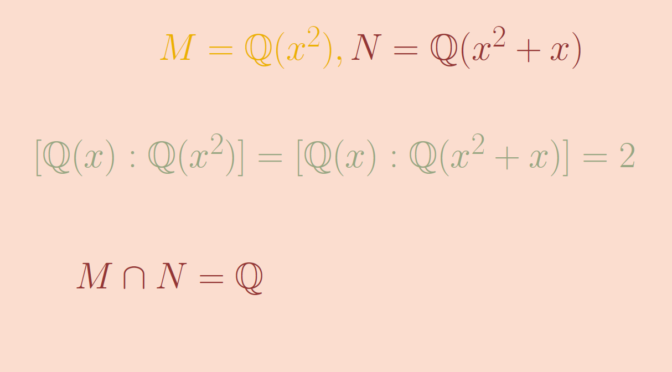# Field not algebraic over an intersection but algebraic over each initial field

Let’s describe an example of a field $$K$$ which is of degree $$2$$ over two distinct subfields $$M$$ and $$N$$ respectively, but not algebraic over $$M \cap N$$.

Let $$K=F(x)$$ be the rational function field over a field $$F$$ of characteristic $$0$$, $$M=F(x^2)$$ and $$N=F(x^2+x)$$. I claim that those fields provide the example we’re looking for.

### $$K$$ is of degree $$2$$ over $$M$$ and $$N$$

The polynomial $$\mu_M(t)=t^2-x^2$$ belongs to $$M[t]$$ and $$x \in K$$ is a root of $$\mu_M$$. Also, $$\mu_M$$ is irreducible over $$M=F(x^2)$$. If that wasn’t the case, $$\mu_M$$ would have a root in $$F(x^2)$$ and there would exist two polynomials $$p,q \in F[t]$$ such that $p^2(x^2) = x^2 q^2(x^2)$ which cannot be, as can be seen considering the degrees of the polynomials of left and right hand sides. This proves that $$[K:M]=2$$. Considering the polynomial $$\mu_N(t)=t^2-t-(x^2+x)$$, one can prove that we also have $$[K:N]=2$$.

### We have $$M \cap N=F$$

The mapping $$\sigma_M : x \mapsto -x$$ extends uniquely to an $$F$$-automorphism of $$K$$ and the elements of $$M$$ are fixed under $$\sigma_M$$. Similarly, the mapping $$\sigma_N : x \mapsto -x-1$$ extends uniquely to an $$F$$-automorphism of $$K$$ and the elements of $$N$$ are fixed under $$\sigma_N$$. Also $(\sigma_N\circ\sigma_M)(x)=\sigma_N(\sigma_M(x))=\sigma_N(-x)=-(-x-1)=x+1.$ An element $$z=p(x)/q(x) \in M \cap N$$ where $$p(x),q(x)$$ are coprime polynomials of $$K=F(x)$$ is fixed under $$\sigma_M \circ \sigma_N$$. Therefore following equality holds $\frac{p(x)}{q(x)}=z=(\sigma_2\circ\sigma_1)(z)=\frac{p(x+1)}{q(x+1)},$ which is equivalent to $p(x)q(x+1)=p(x+1)q(x).$ By induction, we get for $$n \in \mathbb Z$$ $p(x)q(x+n)=p(x+n)q(x).$ Assume $$p(x)$$ is not a constant polynomial. Then it has a root $$\alpha$$ in some finite extension $$E$$ of $$F$$. As $$p(x),q(x)$$ are coprime polynomials, $$q(\alpha) \neq 0$$. Consequently $$p(\alpha+n)=0$$ for all $$n \in \mathbb Z$$ and the elements $$\alpha +n$$ are all distinct as the characteristic of $$F$$ is supposed to be non zero. This implies that $$p(x)$$ is the zero polynomial, in contradiction with our assumption. Therefore $$p(x)$$ is a constant polynomial and $$q(x)$$ also according to a similar proof. Hence $$z$$ is constant as was supposed to be proven.

Finally, $$K=F(x)$$ is not algebraic over $$F=M \cap N$$ as $$(1,x, x^2, \dots, x^n, \dots)$$ is independent over the field $$F$$ which concludes our claims on $$K, M$$ and $$N$$.